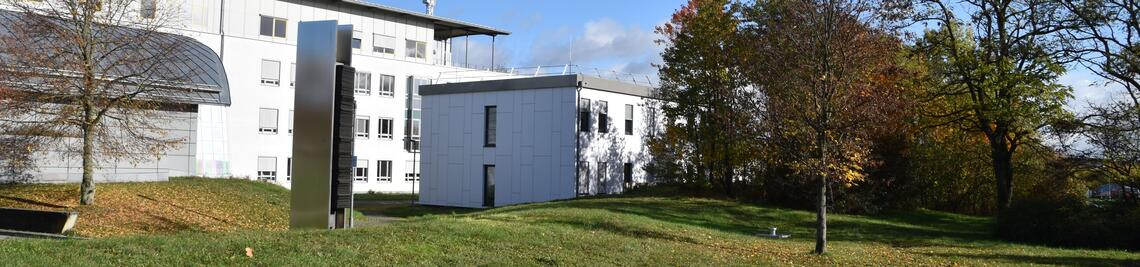•# Drawing Graphs: Geometric Aspects Beyond Planarity

In this project we want to investigate drawing graphs with low visual complexity. By visual complexity we mean the number of geometric primitives needed to represent the graph; for example, slopes, line segments or circular arcs, lines or circles, and, in 3-space, planes or spheres. At the same time, we want to focus on geometric aspects of the recent “beyond planarity” direction in graph drawing by either finding ways to “tame” crossings in the plane or by extending the current research to 3-space.

Consider, for example, the segment number, which is the minimum number of line segments whose union contains a straight-line drawing of a given graph. This measurement for the visual complexity of a graph has already been studied quite extensively for planar graphs. But what about 1-planar graphs, that is, graphs that can be drawn such that each edge can cross at most one other edge? If we know that such a graph actually has a straight-line drawing with at most one crossing per edge, can we non-trivially bound the number of line segments that we need for such a drawing? Can we get better bounds in 3-space, where crossings are not much of an issue?

The plane cover number that we introduced recently serves as a showcase example, too: every planar graph has a crossing-free straight-line drawing in the plane. If we leave, however, the realm of planar graphs, how many planes do we need to accommodate a crossing-free straight-line drawing of a given graph? Consider almost-planar graphs, that is, graphs that becomes planar after the removal of a single edge. While crossing minimization is NP-hard even for almost-planar graphs, we know that we can draw any almost-planar graph on the union of four planes (or, trivially, two spheres). There are many exciting open questions in this area. For example, does a sublinear number of planes suffice for cubic graphs?

 Project Title: Drawing Graphs: Geometric Aspects Beyond Planarity Researchers: Alexander Wolff (local PI), Alexander Ravsky (National Academy of the Sciences, Ukraine) Funding: Deutsche Forschungsgemeinschaft (DFG), in cooperation with SFFRU, Ukraine Term: 2019–2022